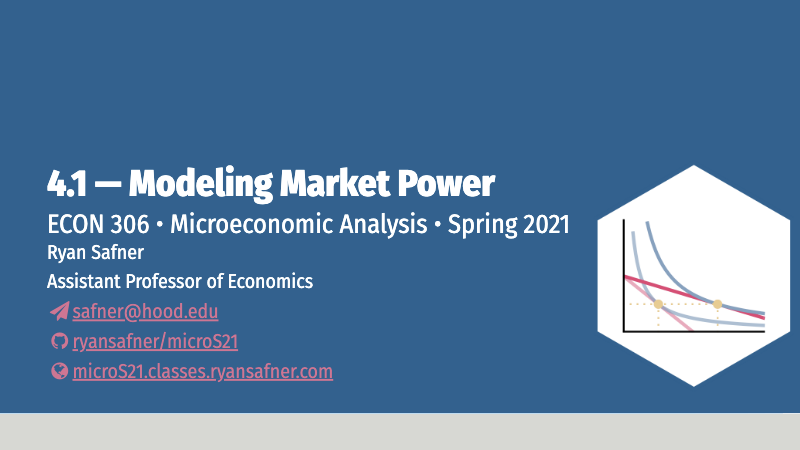# 4.1 — Modeling Firms With Market Power — Class Content

## Contents

• Section 1: Monday, April 19, 2021
• Section 2: Tuesday, April 27, 2021Section 2, because of no class on Thursday Feb 18 and Tuesday Mar 9, and no class on Wednesday April 21, is 1 class behind Section 1.

## Overview

Today we begin our look at “imperfect competition,” where firms have market power, meaning they can charge $$p>MC$$ and search for the profit-maximizing quantity and price. Today is merely about how do change the model to understand how a firm with market power behaves. To assist us, we begin with an extreme case of a single seller, i.e. a monopoly. For now we only assume that there is a single firm, and see how it behaves differently than if it were in a competitive market. Next class we will begin to explore what could cause a market to have only a single seller, and what are some of the social consequences of market power.

• Ch. 9-2-9.5 in @textbook

## Slides## Practice

Today we will be working on practice problems. Answers will be posted later on that page.

## Assignments

### Problem Set 5 Due Sun Apr 25

Problem set 5 (on 3.1-3.5) is due by 11:59 PM Sunday April 25 (both sections) by PDF upload to Blackboard Assignments. This will be your final graded problem set this semester.

## Appendix

### Monopolists Only Produce Where Demand is Elastic: Proof

Let’s first show the relationship between $$MR(q)$$ and price elasticity of demand, $$\epsilon_D$$.

\begin{align*} MR(q) &= p+\bigg(\frac{\Delta p}{\Delta q}\bigg)q && \text{Definition of } MR(q)\\ \frac{MR(q)}{p} &= \frac{p}{p}+\bigg(\frac{\Delta p}{\Delta q}\bigg) \frac{q}{p} && \text{Dividing both sides by } p\\ \frac{MR(q)}{p} &= 1+\underbrace{\bigg(\frac{\Delta p}{\Delta q}\times \frac{q}{p} \bigg)}_{\frac{1}{\epsilon}} && \text{Simplifying}\\ \frac{MR(q)}{p} &= 1+\frac{1}{\epsilon_D} && \text{Recognize price elasticity } \epsilon_D=\frac{\Delta q}{\Delta p} \times \frac{p}{q}\\ MR(q) &= p\bigg(1+\frac{1}{\epsilon_D}\bigg) && \text{Multiplying both sides by }p\\ \end{align*}

Remember, we’ve simplified $$\epsilon_D = \frac{1}{slope} \times \frac{p}{q}$$, where $$\frac{1}{slope} = \frac{\Delta q}{\Delta p}$$ because on a demand curve, $$slope = \frac{\Delta p}{\Delta q}$$.

Now that we have this alternate expression for $$MR(q)$$, lets assume $$MC(q) \geq 0$$ and set them equal to one another to maximize profits:

\begin{align*} MR(q) &= MC(q)\\ p(1+\frac{1}{\epsilon_D}) & = MC(q)\\ p(1-\frac{1}{|\epsilon_D|}) & = MC(q)\\ \end{align*}

I rearrange the last line only to remind us that $$\epsilon_D$$ is always negative!

Now note the following:

• If $$|\epsilon_D| < 1$$, then $$MR(q)$$ is negative. Since $$MC(q)$$ is assumed to be positive, it cannot equal a negative $$MR(q)$$, hence this is not profit-maximizing.
• If $$|\epsilon_D| = 1$$, then $$MR(q)$$ is 0. Only if $$MC(q)$$ is also 0 is this profit-maximizing.
• If $$|\epsilon_D| > 1$$, then $$MR(q)$$ is positive. It can equal a positive $$MC(q)$$ to be profit-maximizing.

Hence, a monopolist will never produce in the inelastic region of the demand curve (where $$MR(q)<0)$$, and will only produce at the unit elastic part of the demand curve (where $$MR(q)=0)$$ if $$MC(q)=0$$. Thus, it generally produces in the elastic region where $$MR(q)>0$$.

See the graphs on slide 31.

### Derivation of the Lerner Index

Marginal revenue is strongly related to the price elasticity of demand, which is $$E_{D}=\frac{\Delta q}{\Delta p} \times \frac{p}{q}$$I sometimes simplify it as $$E_{D}=\frac{1}{slope} \times \frac{p}{q}$$, where “slope” is the slope of the inverse demand curve (graph), since the slope is $$\frac{\Delta p}{\Delta q} = \frac{rise}{run}$$.

We derived marginal revenue (in the slides) as: $MR(q)=p+\frac{\Delta p}{\Delta q}q$

Firms will always maximize profits where:

\begin{align*} MR(q)&=MC(q) && \text{Profit-max output}\\ p+\bigg(\frac{\Delta p}{\Delta q}\bigg)q&=MC(q) && \text{Definition of } MR(q)\\ p+\bigg(\frac{\Delta p}{\Delta q}\bigg) q \times \frac{p}{p}&=MC(q) && \text{Multiplying the left by } \frac{p}{p} \text{ (i.e. 1)}\\ p+\underbrace{\bigg(\frac{\Delta p}{\Delta q}\times \frac{q}{p} \bigg)}_{\frac{1}{\epsilon}} \times p &=MC(q) && \text{Rearranging the left}\\ p+\frac{1}{\epsilon} \times p &=MC(q) && \text{Recognize price elasticity } \epsilon=\frac{\Delta q}{\Delta p} \times \frac{p}{q}\\ p &=MC(q) - \frac{1}{\epsilon} p && \text{Subtract }\frac{1}{\epsilon}p \text{ from both sides}\\ p-MC(q) &= -\frac{1}{\epsilon} p && \text{Subtract }MC(q) \text{ from both sides}\\ \frac{p-MC(q)}{p} &= -\frac{1}{\epsilon} && \text{Divide both sides by }p\\ \end{align*}

The left side gives us the fraction of price that is markup $$\left(\frac{p-MC(q)}{p}\right)$$, and the right side shows this is inversely related to price elasticity of demand.